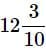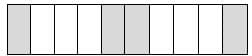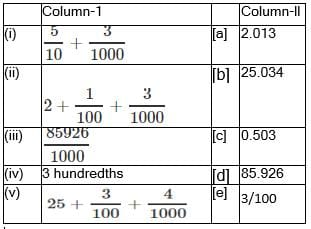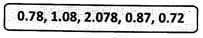## 20 Questions MCQ Test Mathematics (Maths) Class 6 | Olympiad Test: Decimals

Description
Attempt Olympiad Test: Decimals | 20 questions in 20 minutes | Mock test for Class 6 preparation | Free important questions MCQ to study Mathematics (Maths) Class 6 for Class 6 Exam | Download free PDF with solutions
QUESTION: 1

Solution:
QUESTION: 2

Solution:
QUESTION: 3

### What is the decimal form of?

Solution:
QUESTION: 4

What is the fractional form of 46.3?

Solution:
QUESTION: 5

What is the sum of 8/10 + 0 + 7/1000?

Solution:

8/10 + 0 + 7/1000

= 8/10 + 7/1000

= (800 + 7)/1000

= 807/1000

= *0.807* - Ans

QUESTION: 6

Write 4/20 as a decimal.

Solution:
QUESTION: 7

Which of the following is the place value of 3 in 4.375?

Solution:
QUESTION: 8

What is the decimal fraction shown by the shaded part of the figure given?Solution:
QUESTION: 9

7.8 = 7+ 8/?. Which number should replace the ? to make it true?

Solution:
QUESTION: 10

Match the following.Solution:
QUESTION: 11

In which figure does the shaded part represent 0.3?

Solution:
QUESTION: 12

Which of the following options gives the equivalent decimal of 0.08?

Solution:

The equivalent decimal of 0.08 is 0.080.

QUESTION: 13

Which number is greater than 0.08?

Solution:

⇒ 0.7>0.08

QUESTION: 14

Which decimal number is the same as 1/2?

Solution:
QUESTION: 15

Lakshmi is 150 cm tall. What is her height in metres?

Solution:

150 cm = 150/100 = 1.5m

QUESTION: 16

On which side is the place value chart extended to provide place for fractions?

Solution:

Fractions are to the right of ones place in the place value chart.

QUESTION: 17

How does the value of a digit change as it moves from the right to the left in a place value chart?

Solution:

The place value of a digit increases by 10 times as it moves from right to left in a place value chart.

QUESTION: 18

Identify the largest decimal.Solution:

2.0781.080.870.780.72| Hence, 2.078 is the required largest decimal.

QUESTION: 19

What is the expanded form of 78.059?

Solution:

78.059 = 7×10+8×1+0× 1/10 + 5 x 1/100 + 9 x 1/1000

QUESTION: 20

What is the sum of 0.3, 0.03 and 0.003?

Solution:Use Code STAYHOME200 and get INR 200 additional OFF Use Coupon Code